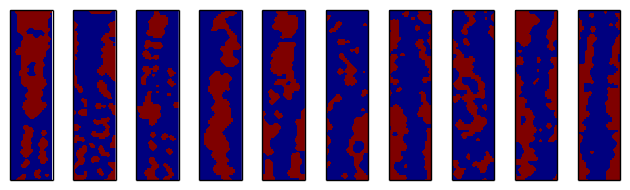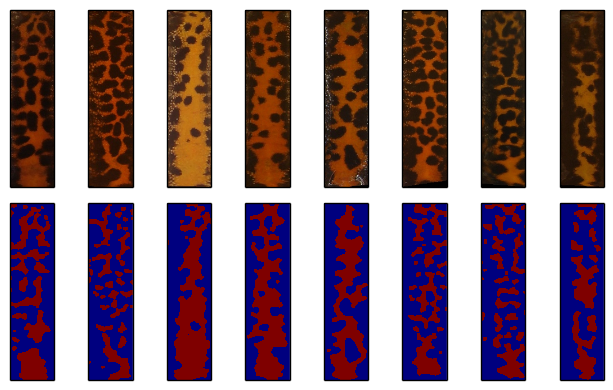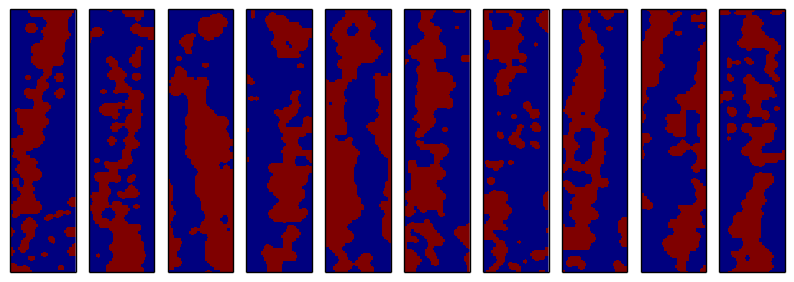# Artificial Spot Patterns for Performance Analysis of Individual Identification of Animals

To assess the identification performance of pattern recognition for wildlife, a reliable ground truth is absolutely necessary. However, often for field work, no ground truth is available or it can only be obtained by manual comparison with high efforts. This would render the usage of automatic photo-identification of wildlife useless.

Alternatively, it can be possible to artificially generate patterns of the species under test by means of statistical algorithms. Then, by generating several patterns for the same individual, a ground truth of arbitrary size can be obtained. These algorithms are trained by a database of unknown patterns and model the statistical properties of this database. Thereafter, in the recreational phase, new patterns that follow the same statistics are obtained by randomly sampling patterns from the model.

In this article, we investigate the ability of Markov chains to reflect the statistics of a database that consists of roughly 6000 patterns of the Great Crested Newt Triturus cristatus.

We can understand each binary pattern as a vector consisting of ones and zeros. The dimension of the vector is equal to the number of pixels in the pattern. Now, understanding the pixels in the pattern as random variables, we can make the assumption, that the distribution of a given pixel only depends on the $N$ previous pixels. Then, we can learn the probability distribution of a pixel given its $N$ previous pixels from the given database.

As an example, consider that the database consists of patters that have relatively large spots, of diameter of 50px on average. Now, if the previous 10 pixels where set to zero (i.e. dark spot), it is very likely, that the following pixel will also be zero (because spots are 50px on average). On the other hand, if the previous 100 pixels where zero, it is more likely that the next pixel becomes one, since the spot is likely to end soon. Once we know the distribution of one pixel, we can draw a sample from its distribution and use it as the value for this pixel. Subsequently, the next pixel can be calculated. A more detailed description of the technique can be found in this article, which was used to reproduce language that follows a given model.

In the following picture, we present some artificially generated patterns with this method:

N = 10
figure(figsize=(6.5,2))
for i in range(N):
subplot(1,N,i+1)
Im_full = createPattern()
imshow(Im_full, interpolation='none'); xticks([]); yticks([]);
plt.tight_layout()For comparison, here are some patterns of the database, along with their binary representation:

imgInds = [1, 10, 51, 55, 156, 190, 202, 210]
files = glob.glob(baseDir + '*.jpg')
el = cv2.getStructuringElement(cv2.MORPH_ELLIPSE, (6,6))
figure(figsize=(8,4))
for i, ind in enumerate(imgInds):
BW = cv2.morphologyEx(ai.thresh_BasedOnMaximumOfAverage(img), cv2.MORPH_OPEN, el)

subplot(2, N, 1+i); imshow(img); xticks([]); yticks([])
subplot(2, N, 1+N+i); imshow(BW, interpolation='none'); xticks([]); yticks([]);
plt.tight_layout()#ignore
import numpy as np
import sys, os
import glob
import cv2
import matplotlib.pyplot as plt

sys.path.append("/home/mmatthe/temp/builds/ai/src/PythonExtensions")
import amphident as ai

%matplotlib inline
from pylab import *


Provide the directory where the patterns are located and set up the size for the patterns. Here, we use patterns of size 20 times 80 pixel.

baseDir = '/home/mmatthe/programming/AmphIdent_misc/newts/KMData/'
size = 80*320
sizeT = (80, 320)
downScale = 4

size = size / (downScale * downScale)
sizeT = tuple(x/downScale for x in sizeT)


Load all the patterns in the database and convert them to a binary representation. Finally, join all textual representations of the patterns together, separeted by a separator text.

files = glob.glob(baseDir + '*.jpg')

el = cv2.getStructuringElement(cv2.MORPH_ELLIPSE, (3,3))
count = len(files)
patStart = 4*sizeT
data = np.zeros((count, patStart+size), dtype=np.uint8)
for i, f in enumerate(files):
imgR = cv2.resize(img, sizeT)
BW = ai.thresh_BasedOnMaximumOfAverage(imgR)
BW = cv2.morphologyEx(BW, cv2.MORPH_OPEN, el)
BW /= 255
data[i,:patStart] = 2
data[i,patStart:] = BW.flatten()
data_str = "".join("%d" % x for x in data.flatten())


The below function learns the probability distribution given the order of the Markov chain.

from collections import *

def train_char_lm(data, order=4):
lm = defaultdict(Counter)
for i in xrange(len(data)-order):
history, char = data[i:i+order], data[i+order]
lm[history][char]+=1
def normalize(counter):
s = float(sum(counter.values()))
return [(c,cnt/s) for c,cnt in counter.iteritems()]
outlm = {hist:normalize(chars) for hist, chars in lm.iteritems()}
return outlm


Train our model with an order of 60. I.e. the model should consider the values of the 60 previous pixels, to determine the distribution of the following pixel.

O60 = train_char_lm(data_str, order=60)


The functions to generate a letter based on the model, and to sample a text from a given model.

from random import random

def generate_letter(lm, history, order):
history = history[-order:]
dist = lm[history]
x = random()
for c,v in dist:
x = x - v
if x <= 0: return c

def generate_text(lm, order, nletters=1000):
history = "~" * order
out = []
for i in xrange(nletters):
c = generate_letter(lm, history, order)
history = history[-order:] + c
out.append(c)
return "".join(out)


The function to tranform a text to an image. We generate a pattern that is of double height, however we use a random portion from its middle, to mitigate the effects of the sampling reaching the steady state.

def txtToIm(text):
ints = [int(x) for x in text if x != "2"]
rows = len(ints) / sizeT
ints = ints[:(rows*sizeT)]
BW = np.array(ints).reshape((rows, sizeT))
BW = cv2.resize(BW.astype(np.uint8), (80, rows*downScale))
el = cv2.getStructuringElement(cv2.MORPH_ELLIPSE, (5,5))
BW = cv2.morphologyEx(BW, cv2.MORPH_OPEN, el)
return BW

def createPattern():
Im = txtToIm(generate_text(O60, 60, nletters=2*size))
starty = np.random.randint(Im.shape-320)
Im_full = Im[starty:(starty+320), :]
return Im_full



Finally, we can use the model to generate some random patterns.

N = 10
figure(figsize=(10,4))
for i in range(N):
subplot(1,N,i+1)
Im_full = createPattern()
imshow(Im_full, interpolation='none'); xticks([]); yticks([]);As can be seen, the patterns have the same visual appearance as the patterns from the database. However, looking at the pattern quality and comparing it to the original patterns in the beginning, they are not fully accurate. In particular, the patterns occur slanted or the read area is not centered in the middle. This is due to the fact that the markov chain actually has no information about the position of the current pixel. So, it can happen that the overall process drifts to one side of the pattern. This can be mitigated by providing positional information to the chain as an extra parameter or increasing the model memory.

By generating several patterns, the database size can be increased to challenge the algorithm. On the other hand, when taking one pattern and slightly modifying it according to the impairments that occur in the photographing session (small posture change, dirt, sharpness, etc), the pattern matching algorithm can be thoroughly tested with a large, artificially generated database.

Mo, 16 Mai 2016 - Maximilian Matthe